# NCERT Solutions for Class 10th: Ch 12 Electricity Science

## NCERT Solutions for Class 10th: Ch 12 Electricity Science

In Text Questions

Page No: 200

1. What does an electric circuit mean?

Answer

A continuous and closed path of an electric current is called an electric circuit. An electric circuit consists of electric devices, source of electricity and wires that are connected with the help of a switch.

2. Define the unit of current.

Answer

The unit of electric current is ampere (A). 1 A is defined as the flow of 1 C of charge through a wire in 1 s.

3. Calculate the number of electrons constituting one coulomb of charge.

Answer

One electron possesses a charge of 1.6 ×10-19C, i.e., 1.6 ×10-19C of charge is contained in 1 electron.
∴ 1 C of charge is contained in 1/1.6 x 10-19 = 6.25 x 1018 = 6 x 1018
Therefore, 6 x 1018 electrons constitute one coulomb of charge.

Page No: 202

1. Name a device that helps to maintain a potential difference across a conductor.

Answer

Any source of electricity like battery, cell, power supply, etc. helps to maintain a potential difference across a conductor.

2. What is meant by saying that the potential difference between two points is 1 V?

Answer

If 1 J of work is required to move a charge of amount 1 C from one point to another, then it is said that the potential difference between the two points is 1 V.

3. How much energy is given to each coulomb of charge passing through a 6 V battery?

Answer

The energy given to each coulomb of charge is equal to the amount of work which is done in moving it.
Now we know that,
Potential difference = Work Done/Charge
∴ Work done = Potential difference × charge
Where, Charge = 1 C and Potential difference = 6 V
∴ Work done = 6×1
= 6 Joule.

Page No: 209

1. On what factors does the resistance of a conductor depend?

Answer

The resistance of a conductor depends upon the following factors:
→ Length of the conductor
→ Cross-sectional area of the conductor
→ Material of the conductor
→ Temperature of the conductor

2. Will current flow more easily through a thick wire or a thin wire of the same material, when connected to the same source? Why?

Answer

The current will flow more easily through thick wire. It is because the resistance of a
conductor is inversely proportional to its area of cross - section. If thicker the wire, less is resistance and hence more easily the current flows.

3. Let the resistance of an electrical component remains constant while the potential difference across the two ends of the component decreases to half of its former value. What change will occur in the current through it?

Answer

According to Ohm’s law
V = IR
I=V/R ...                   (1)
Now Potential difference is decreased to half
∴ New potential difference =V/2
Resistance remains constant
So the new current = Vʹ/R
= (V/2)/R
= (1/2) (V/R)
= (1/2) I = I/2
Therefore, the amount of current flowing through the electrical component is reduced by half.

4. Why are coils of electric toasters and electric irons made of an alloy rather than a pure metal?

Answer

The resistivity of an alloy is higher than the pure metal. Moreover, at high temperatures, the alloys do not melt readily. Hence, the coils of heating appliances such as electric toasters and electric irons are made of an alloy rather than a pure metal.

5. Use the data in Table 12.2 to answer the following -
Table 12.2 Electrical resistivity of some substances at 20°C
 − Material Resistivity (Ω m) Conductors Silver 1.60 × 10−8 Copper 1.62 × 10−8 Aluminium 2.63 × 10−8 Tungsten 5.20 × 10−8 Nickel 6.84 × 10−8 Iron 10.0 × 10−8 Chromium 12.9 × 10−8 Mercury 94.0 × 10−8 Manganese 1.84 × 10−6 Constantan (alloy of Cu and Ni) 49 × 10−6 Alloys Manganin (alloy of Cu, Mn and Ni) 44 × 10−6 Nichrome (alloy of Ni, Cr, Mn and Fe) 100 × 10−6 Glass 1010 − 1014 Insulators Hard rubber 1013 − 1016 Ebonite 1015 − 1017 Diamond 1012 − 1013 Paper (dry) 1012

Answer

(a) Resistivity of iron = 10.0 x 10-8 Ω
Resistivity of mercury = 94.0 x 10-8 Ω
Resistivity of mercury is more than that of iron. This implies that iron is a better conductor than mercury.

(b) It can be observed from Table 12.2 that the resistivity of silver is the lowest among the listed materials. Hence, it is the best conductor.

Page No: 213

1. Draw a schematic diagram of a circuit consisting of a battery of three cells of 2 V each, a 5 Ω resistor, an 8 Ω resistor, and a 12 Ω resistor, and a plug key, all connected in series.

Answer

Three cells of potential 2 V, each connected in series therefore the potential difference of the battery will be 2 V + 2 V + 2 V = 6V. The following circuit diagram shows three resistors of resistances 5 Ω, 8 Ω and 12 Ω respectively connected in series and a battery of potential 6 V and a plug key which is closed means the current is flowing in the circuit.

2. Redraw the circuit of question 1, putting in an ammeter to measure the current through the resistors and a voltmeter to measure potential difference across the 12 Ω resistor. What would be the readings in the ammeter and the voltmeter?

Answer

An ammeter should be connected in the circuit in series with the resistors. To measure the potential difference across the resistor it should be connected in parallel, as shown in the following figure.The resistances are connected in series.
Ohm’s law can be used to obtain the readings of ammeter and voltmeter. According to Ohm’s law,
V = IR,
Where,
Potential difference, V = 6 V
Current flowing through the circuit/resistors = I
Resistance of the circuit, R = 5 + 8 + 12 = 25Ω
I = V/R = 6/25 = 0.24 A
Potential difference across 12 Ω resistor = V1
Current flowing through the 12 Ω resistor, I = 0.24 A
Therefore, using Ohm’s law, we obtain
V1 = IR = 0.24 x 12 = 2.88 V
Therefore, the reading of the ammeter will be 0.24 A.
The reading of the voltmeter will be 2.88 V.

Page No: 216

1. Judge the equivalent resistance when the following are connected in parallel − (a) 1 Ω and 106Ω, (b) 1 Ω and 103Ω and 106Ω.

Answer

(a) When 1 Ω and 106 Ω are connected in parallel:
Let R be the equivalent resistance.Therefore, equivalent resistance ≈ 1 Ω

(b) When 1Ω, 103 Ω and 106 Ω are connected in parallel:
Let R be the equivalent resistance.Therefore, equivalent resistance = 0.999 Ω

2. An electric lamp of 100 Ω, a toaster of resistance 50 Ω, and a water filter of resistance 500 Ω are connected in parallel to a 220 V source. What is the resistance of an electric iron connected to the same source that takes as much current as all three appliances, and what is the current through it?

Answer

Resistance of electric lamp, R1 = 100 Ω
Resistance of toaster, R2 = 50 Ω
Resistance of water filter, R3 = 500 Ω
Potential difference of the source, V = 220 V
These are connected in parallel, as shown in the following figure.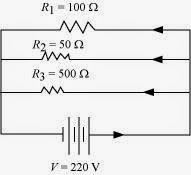Let R be the equivalent resistance of the circuit.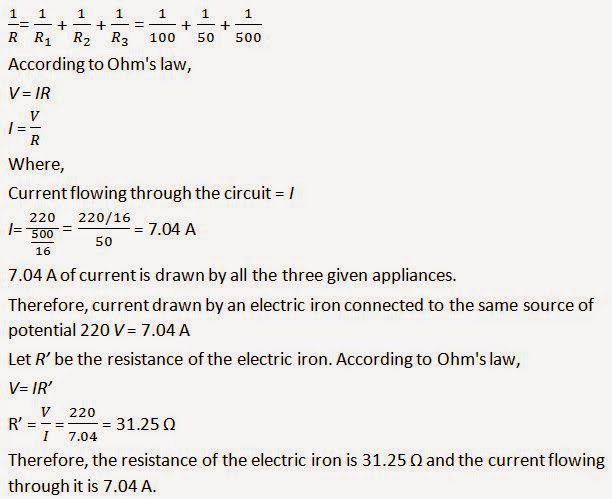3. What are the advantages of connecting electrical devices in parallel with the battery instead of connecting them in series?

Answer

There is no division of voltage among the appliances when connected in parallel. The potential difference across each appliance is equal to the supplied voltage.
The total effective resistance of the circuit can be reduced by connecting electrical appliances in parallel.

4. How can three resistors of resistances 2 Ω, 3 Ω and 6 Ω be connected to give a total resistance of (a) 4 Ω, (b) 1 Ω?

Answer

There are three resistors of resistances 2 Ω, 3 Ω, and 6 Ω respectively.

(a) The following circuit diagram shows the connection of the three resistors.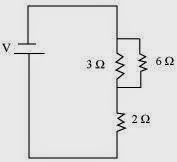Here, 6 Ω and 3 Ω resistors are connected in parallel.
Therefore, their equivalent resistance will be given byThis equivalent resistor of resistance 2 Ω is connected to a 2 Ω resistor in series.
Therefore, the equivalent resistance of the circuit = 2 Ω + 2 Ω = 4 Ω
Hence the total resistance of the circuit is 4 Ω.

(b) The following circuit diagram shows the connection of the three resistors.All the resistors are connected in series. Therefore, their equivalent resistance will be given as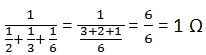Therefore, the total resistance of the circuit is 1 Ω.

5. What is (a) the highest, (b) the lowest total resistance that can be secured by combinations of four coils of resistance 4 Ω, 8 Ω, 12 Ω, 24 Ω?

Answer

There are four coils of resistances 4 Ω, 8 Ω, 12 Ω and 24 Ω respectively.

(a) If these coils are connected in series, then the equivalent resistance will be the highest, given by the sum 4 + 8 + 12 + 24 = 48 Ω

(b) If these coils are connected in parallel, then the equivalent resistance will be the lowest, given byTherefore, 2 Ω is the lowest total resistance.

Page No: 218

1. Why does the cord of an electric heater not glow while the heating element does?

Answer

The heating element of the heater is made up of alloy which has very high resistance so when current flows through the heating element, it becomes too hot and glows red. But the resistance of cord which is usually of copper or aluminium is very law so it does not glow.

2. Compute the heat generated while transferring 96000 coulomb of charge in one hour through a potential difference of 50 V.

Answer

Given Charge, Q = 96000C
Time, t= 1hr = 60 x 60= 3600s
Potential difference, V= 50volts
Now we know that H= VIt
So we have to calculate I first
As I= Q/t
∴ I = 96000/3600 = 80/3 A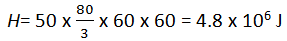Therefore, the heat generated is 4.8 x 106 J.

3. An electric iron of resistance 20 Ω takes a current of 5 A. Calculate the heat developed in 30 s.

Answer

The amount of heat (H) produced is given by the joule's law of heating asH= Vlt
Where,
Current, I = 5 A
Time, t = 30 s
Voltage, V = Current x Resistance = 5 x 20 = 100 V
H= 100 x 5 x 30 = 1.5 x 104 J.

Therefore, the amount of heat developed in the electric iron is 1.5 x 104 J.

Page No: 220

1. What determines the rate at which energy is delivered by a current?

Answer

The rate of consumption of electric energy in an electric appliance is called electric power. Hence, the rate at which energy is delivered by a current is the power of the appliance.

2. An electric motor takes 5 A from a 220 V line. Determine the power of the motor and the energy consumed in 2 h.

Answer

Power (P) is given by the expression,P = VI
Where,
Voltage,V = 220 V
Current, I = 5 A
P= 220 x 5 = 1100 W
Energy consumed by the motor = Pt
Where,
Time, t = 2 h = 2 x 60 x 60 = 7200 s
P = 1100 x 7200 = 7.92 x 106 J
Therefore, power of the motor = 1100 W
Energy consumed by the motor = 7.92 x 106 J

Page No: 221

Exercise

1. A piece of wire of resistance R is cut into five equal parts. These parts are then connected in parallel. If the equivalent resistance of this combination is R', then the ratio R/R' is -(a) 1/25
(b) 1/5
(c) 5
(d) 25
► (d) 25

2. Which of the following terms does not represent electrical power in a circuit?
(a) I2R
(b) IR2
(c) VI
(d) V2/R
► (b) IR2

3. An electric bulb is rated 220 V and 100 W. When it is operated on 110 V, the power consumed will be -
(a) 100 W
(b) 75 W
(c) 50 W
(d) 25 W
► (d) 25 W

4. Two conducting wires of the same material and of equal lengths and equal diameters are first connected in series and then parallel in a circuit across the same potential difference. The ratio of heat produced in series and parallel combinations would be -
(a) 1:2
(b) 2:1
(c) 1:4
(d) 4:1
► (c) 1:4

5. How is a voltmeter connected in the circuit to measure the potential difference between two points?

Answer

To measure the potential difference between two points, a voltmeter should be connected in parallel to the points.

6. A copper wire has diameter 0.5 mm and resistivity of 1.6 × 10−8 Ω m. What will be the length of this wire to make its resistance 10 Ω? How much does the resistance change if the diameter is doubled?

Answer

Area of cross-section of the wire, A =π (d/2) 2
Diameter= 0.5 mm = 0.0005 m
Resistance, R = 10 Ω
We know thatTherefore, the length of the wire is 122.7 m and the new resistance is 2.5 Ω.

7. The values of current I flowing in a given resistor for the corresponding values of potential difference V across the resistor are given below −
 I (amperes ) 0.5 1 2 3 4 V (volts) 1.6 3.4 6.7 10.2 13.2
Plot a graph between V and I and calculate the resistance of that resistor.

Answer

The plot between voltage and current is called IV characteristic. The voltage is plotted on x-axis and current is plotted on y-axis. The values of the current for different values of the voltage are shown in the given table.

 V (volts) 1.6 3.4 6.7 10.2 13.2 I (amperes ) 0.5 1 2 3 4

The IV characteristic of the given resistor is plotted in the following figure.The slope of the line gives the value of resistance (R) as,
Slope = 1/R = BC/AC = 2/6.8
R= 6.8/2 = 3.4 Ω
Therefore, the resistance of the resistor is 3.4 Ω.

8. When a 12 V battery is connected across an unknown resistor, there is a current of 2.5 mA in the circuit. Find the value of the resistance of the resistor.

Answer

Resistance (R) of a resistor is given by Ohm's law as,V= IR
R= V/I
Where,
Potential difference, V= 12 V
Current in the circuit, I= 2.5 mA = 2.5 x 10-3 ATherefore, the resistance of the resistor is 4.8 kΩ

9. A battery of 9 V is connected in series with resistors of 0.2 Ω, 0.3 Ω, 0.4 Ω, 0.5 Ω and 12 Ω, respectively. How much current would flow through the 12 Ω resistor?

Answer

There is no current division occurring in a series circuit. Current flow through the component is the same, given by Ohm’s law as
V= IR
I= V/R
Where,
R is the equivalent resistance of resistances 0.2 Ω, 0.3 Ω, 0.4 Ω, 0.5 Ω and 12 Ω. These are connected in series. Hence, the sum of the resistances will give the value of R.
R= 0.2 + 0.3 + 0.4 + 0.5 + 12 = 13.4 Ω
Potential difference, V= 9 V
I= 9/13.4 = 0.671 A
Therefore, the current that would flow through the 12 Ω resistor is 0.671 A.

10. How many 176 Ω resistors (in parallel) are required to carry 5 A on a 220 V line?

Answer

For x number of resistors of resistance 176 Ω, the equivalent resistance of the resistors connected in parallel is given by Ohm's law asV= IR
R= V/I
Where,
Supply voltage, V= 220 V
Current, I = 5 A
Equivalent resistance of the combination = R,given asTherefore, four resistors of 176 Ω are required to draw the given amount of current.

11. Show how you would connect three resistors, each of resistance 6 Ω, so that the combination has a resistance of (i) 9 Ω, (ii) 4 Ω.

Answer

If we connect the resistors in series, then the equivalent resistance will be the sum of the resistors, i.e., 6 Ω + 6 Ω + 6 Ω = 18 Ω, which is not desired. If we connect the resistors in parallel, then the equivalent resistance will be 6/2 = 3 Ω is also not desired. Hence, we should either connect the two resistors in series or parallel.
(a) Two resistor in parallelTwo 6 Ω resistors are connected in parallel. Their equivalent resistance will be

The third 6 Ω resistor is in series with 3 Ω. Hence, the equivalent resistance of the circuit is 6 Ω+ 3 Ω = 9 Ω.
(b) Two resistor in seriesTwo 6 Ω resistors are in series. Their equivalent resistance will be the sum 6 + 6 = 12 Ω.
The third 6 Ω resistor is in parallel with 12 Ω. Hence, equivalent resistance will be

Therefore, the total resistance is 4 Ω.

12. Several electric bulbs designed to be used on a 220 V electric supply line, are rated 10 W. How many lamps can be connected in parallel with each other across the two wires of 220 V line if the maximum allowable current is 5 A?

Answer

Resistance R1 of the bulb is given by the expression,
Supply voltage, V = 220 V
Maximum allowable current, I = 5 A
Rating of an electric bulb P=10watts
Because R=V2/P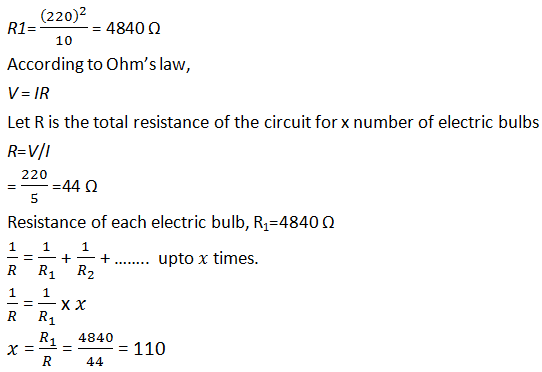∴ Number of electric bulbs connected in parallel are 110.

13. A hot plate of an electric oven connected to a 220 V line has two resistance coils A and B, each of 24 Ω resistances, which may be used separately, in series, or in parallel. What are the currents in the three cases?

Answer

Supply voltage, V= 220 V
Resistance of one coil, R= 24 Ω

(i) Coils are used separately
According to Ohm's law,
V= I1R1
Where,
I1 is the current flowing through the coil
I1 = V/R1 = 220/24 = 9.166 A
Therefore, 9.16 A current will flow through the coil when used separately.

(ii) Coils are connected in series
Total resistance, R2 = 24 Ω + 24 Ω = 48 Ω
According to Ohm's law,V = I2R2
Where,
I2 is the current flowing through the series circuit
I2 = V/R2 = 220/48 = 4.58 A
Therefore, 4.58 A current will flow through the circuit when the coils are connected in series.
(iii) Coils are connected in parallel
Total resistance, R3 is given as =

According to Ohm's law,
V= I3R3
Where,
I3 is the current flowing through the circuit I3 = V/R3 = 220/12 = 18.33 A
Therefore, 18.33 A current will flow through the circuit when coils are connected in parallel.

14. Compare the power used in the 2 Ω resistor in each of the following circuits: (i) a 6 V battery in series with 1 Ω and 2 Ω resistors, and (ii) a 4 V battery in parallel with 12 Ω and 2 Ω resistors.

Answer

(i) Potential difference, V = 6 V
1 Ω and 2 Ω resistors are connected in series. Therefore, equivalent resistance of the circuit, R = 1 + 2 = 3 Ω
According to Ohm’s law,
V = IR
Where,
I is the current through the circuit
I= 6/3 = 2 A
This current will flow through each component of the circuit because there is no division of current in series circuits. Hence, current flowing through the 2 Ω resistor is 2 A. Power is given by the expression,
P= (I)2R = (2)2 x 2 = 8 W

(ii) Potential difference, V = 4 V
12 Ω and 2 Ω resistors are connected in parallel. The voltage across each component of a parallel circuit remains the same. Hence, the voltage across 2 Ω resistor will be 4 V.
Power consumed by 2 Ω resistor is given by
P= V2/R = 42/2 = 8 W
Therefore, the power used by 2 Ω resistor is 8 W.

15. Two lamps, one rated 100 W at 220 V, and the other 60 W at 220 V, are connected in parallel to electric mains supply. What current is drawn from the line if the supply voltage is 220 V?

Answer

Both the bulbs are connected in parallel. Therefore, potential difference across each of them will be 220 V, because no division of voltage occurs in a parallel circuit.
Current drawn by the bulb of rating 100 W is given by,Power = Voltage x Current
Current =  Power/Voltage = 60/220 A
Hence, current drawn from the line = 100/220 + 60/220 = 0.727 A

16. Which uses more energy, a 250 W TV set in 1 hr, or a 1200 W toaster in 10 minutes?

Answer

Energy consumed by an electrical appliance is given by the expression,H= Pt
Where,
Power of the appliance = P
Time = t
Energy consumed by a TV set of power 250 W in 1 h = 250 ×3600 = 9 ×105 J
Energy consumed by a toaster of power 1200 W in 10 minutes = 1200 ×600
Energy consumed by a toaster of power 1200 W in 10 minutes = 1200 ×600
= 7.2×105 J
Therefore, the energy consumed by a 250 W TV set in 1 h is more than the energy consumed by a toaster of power 1200 W in 10 minutes.

17. An electric heater of resistance 8 Ω draws 15 A from the service mains 2 hours. Calculate the rate at which heat is developed in the heater.

Answer

Rate of heat produced by a device is given by the expression for power as, P= I2R
Where,
Resistance of the electric heater, R= 8 Ω
Current drawn, I = 15 A
P= (15)2 x 8 = 1800 J/s
Therefore, heat is produced by the heater at the rate of 1800 J/s.

18. Explain the following.
(a) Why is the tungsten used almost exclusively for filament of electric lamps?
(b) Why are the conductors of electric heating devices, such as bread-toasters and electric irons, made of an alloy rather than a pure metal?
(c) Why is the series arrangement not used for domestic circuits?
(d) How does the resistance of a wire vary with its area of cross-section?
(e) Why are copper and aluminium wires usually employed for electricity transmission?

Answer

(a) The melting point and of Tungsten is an alloy which has very high melting point and very high resistivity so does not burn easily at a high temperature.

(b) The conductors of electric heating devices such as bread toasters and electric irons are made of alloy because resistivity of an alloy is more than that of metals which produces large amount of heat.

(c) In series circuits voltage is divided. Each component of a series circuit receives a small voltage so the amount of current decreases and the device becomes hot and does not work properly. Hence, series arrangement is not used in domestic circuits.

(d) Resistance (R) of a wire is inversely proportional to its area of cross-section (A), i.e. when area of cross section increases the resistance decreases or vice versa.

(e) Copper and aluminium are good conductors of electricity also they have low resistivity. So they are usually used for electricity transmission.

Notes of Electricity

Extra Questions for Electricity

Go Back To NCERT Solutions for Class 10th Science

Watch age fraud in sports in India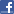Facebook Comments 1 Comments

Watch animated chapter videos and read e-books on premiumstudyrankers app. 55% off for today

© 2019 Study Rankers is a registered trademark.